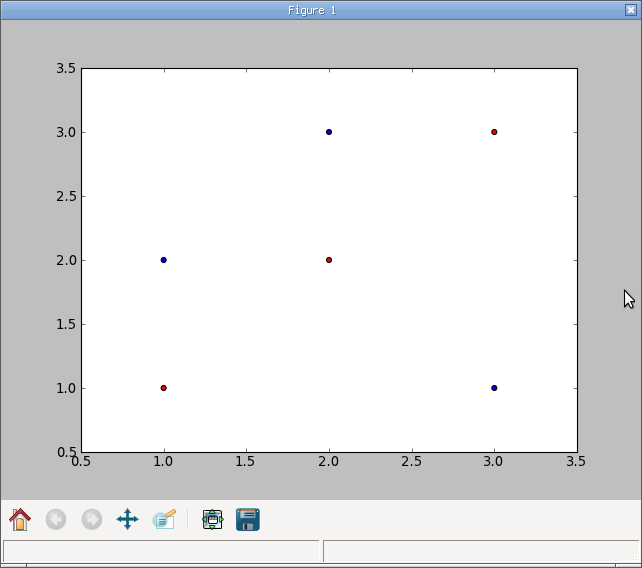# Strange behaviour of scatter with single-column 2D array

Hello,
I've run into a strange behaviour of matplotlib while trying to figure
out why my data was displayed incorrectly.
I'm note quite sure if this is a bug or expected behaviour, but I feel
it's kind of counter-intuitive, so I'm posting here.

The attached python script does a scatter plot of some data. I'm using
the first column as the x coordinates and the second as
y. Looking at the matrix (x == y), I'd expect the three data point to
be on a diagonal line.

Now there seem to be a difference on how numpy handles A[:,0]
depending on if A is a np.array or np.matrix. In the case of
an array, a 1D array is returned, in the case of a matrix, a 2D Nx1
matrix is returned. Using this matrix seems to confuse matplotlib.

Using np.ravel or np.flatten on the slices fix that problem.

Is there an explanation for this behaviour or should I fill a bug ?

Best regards,
Julien Rebetezmatrix_plot.py (396 Bytes)

LJulien Rebetez :

I've run into a strange behaviour of matplotlib while trying to figure
out why my data was displayed incorrectly.
I'm note quite sure if this is a bug or expected behaviour, but I feel
it's kind of counter-intuitive, so I'm posting here.

...
Now there seem to be a difference on how numpy handles A[:,0]
depending on if A is a np.array or np.matrix. In the case of
an array, a 1D array is returned, in the case of a matrix, a 2D Nx1
matrix is returned. Using this matrix seems to confuse matplotlib.

Using np.ravel or np.flatten on the slices fix that problem.

Is there an explanation for this behaviour or should I fill a bug ?

Don't fill a bug.
They explain that a slice of a matrix is a matrix, and its "view" is different from what you get for arrays.

But, no need to reshape the stuff. Just use the "array attribute" of the matrix :

pl.scatter(B.A[:,0], B.A[:,1], c='b')

//Here 'A' is the name of the attribute, nothing to do with your array A; by chance it is the same...//

The best

Jerzy Karczmarczuk

I've read the numpy tutorial and I get it that array and matrices
behave differently.

Now, what I find kind of strange is that the plot I get when directly
feeding the matrices to scatter
doesn't really seem to represent anything.
I think that, if possible, showing an error or a warning would be much
more appropriate than showing
a plot. It would let the user know that the problem is not with her
dataset, but with the plot.

Wouldn't it be possible to simply check the shape in scatter() and
display a warning if it has more than one dimension ?

Best,
Julien

···

On Tue, Mar 20, 2012 at 3:52 PM, Jerzy Karczmarczuk <jerzy.karczmarczuk@...3937...> wrote:

LJulien Rebetez :

I've run into a strange behaviour of matplotlib while trying to figure
out why my data was displayed incorrectly.
I'm note quite sure if this is a bug or expected behaviour, but I feel
it's kind of counter-intuitive, so I'm posting here.

...
Now there seem to be a difference on how numpy handles A[:,0]
depending on if A is a np.array or np.matrix. In the case of
an array, a 1D array is returned, in the case of a matrix, a 2D Nx1
matrix is returned. Using this matrix seems to confuse matplotlib.

Using np.ravel or np.flatten on the slices fix that problem.

Is there an explanation for this behaviour or should I fill a bug ?

Don't fill a bug.
They explain that a slice of a matrix is a matrix, and its "view" is
different from what you get for arrays.

But, no need to reshape the stuff. Just use the "array attribute" of the
matrix :

pl.scatter(B.A[:,0], B.A[:,1], c='b')

//Here 'A' is the name of the attribute, nothing to do with your array
A; by chance it is the same...//

The best

Jerzy Karczmarczuk

------------------------------------------------------------------------------
This SF email is sponsosred by:
http://p.sf.net/sfu/sfd2d-msazure
_______________________________________________
Matplotlib-users mailing list
Matplotlib-users@lists.sourceforge.net
https://lists.sourceforge.net/lists/listinfo/matplotlib-users

I think this is more of an issue that we simply never tested for matrices. As for the shape tests, scatter can take 2d arrays, iirc, with each column being a different color. (again, all from my memory, which would easily fail memcheck).

Ben Root

···

On Tuesday, March 20, 2012, Julien Rebetez <julien.rebetez@…287…> wrote:

I’ve read the numpy tutorial and I get it that array and matrices

behave differently.

Now, what I find kind of strange is that the plot I get when directly
feeding the matrices to scatter
doesn’t really seem to represent anything.
I think that, if possible, showing an error or a warning would be much

more appropriate than showing
a plot. It would let the user know that the problem is not with her
dataset, but with the plot.

Wouldn’t it be possible to simply check the shape in scatter() and

display a warning if it has more than one dimension ?

Best,
Julien